##### Solution of Inhomogeneous Differential Equations with Polynomial Coefficients in Terms of the Green's Function and the AC-Laplace Transform

Tohru Morita, Ken-ichi Sato

Journal of Advances in Mathematics and Computer Science, Page 1-22
DOI: 10.9734/JAMCS/2018/43059

The particular solutions of inhomogeneous differential equations with polynomial coefficients in terms of the Green's function are obtained in the framework of the AC-Laplace transform, that is the Laplace transform supplemented by its analytic continuation. In particular, discussions are given on Kummer's and the hypergeometric differential equation, and also on a fractional differential equation with coefficients of polynomial of at most first degree.

##### Determinant and Inverse of a Skew Peoeplitz Matrix♯

Muen Han, Zhaolin Jiang

Journal of Advances in Mathematics and Computer Science, Page 1-21
DOI: 10.9734/JAMCS/2018/43496

In this paper, we consider the determinant and the inverse of a skew Peoeplitz matrix and a skew Peankel matrix involving Perrin numbers. we first give the definition of a skew Peoeplitz matrix and a skew Peankel matrix. Then we compute the determinant and the inverse of the skew Peoeplitz matrix and the skew Peankel matrix by constructing the transformation matrices.

##### Geometric Series on Fourier Cosine-Sine Transform

Belete Debalkie Mekonen, Getachew Abiye Salilew

Journal of Advances in Mathematics and Computer Science, Page 1-4
DOI: 10.9734/JAMCS/2018/42892

The aim of this study is to provide new properties of geometric series on Fourier cosine and sine transform. The authors also presented very short form of general properties of Fourier cosine and sine transform with a product of a power series at a non-negative real number b in a very elementary ways.

##### On Space-Time Fractional Heat Type Non-Homogeneous Time-Fractional Poisson Equation

Ejighikeme McSylvester Omaba

Journal of Advances in Mathematics and Computer Science, Page 1-18
DOI: 10.9734/JAMCS/2018/33896

Consider the following space-time fractional heat equation with Riemann-Liouville derivative of
non-homogeneous time-fractional Poisson process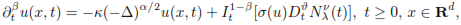where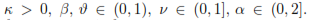The operator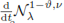with(t) the Riemann-Liouville non-homogeneous fractional integral process,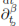is the Caputo fractional derivative,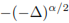is the generator of an isotropic stable process,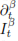is the fractional integral operator, and σ : R → R is Lipschitz continuous. The above time fractional stochastic heat type equations may be used to model sequence of catastrophic events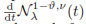for some speciﬁc
rate functions were computed. Consequently, the growth moment bounds for the class of heat equation perturbed with the non-homogeneous fractional time Poisson process were given and we show that the solution grows exponentially for some small time intervaland t0> 1; that is, the result establishes that the energy of the solution grows atleast as c4(t + t0)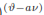exp(c5t) and at most as c1texp(c3t) for different conditions on the initialdata, where c1, c3, c4 and c5  are some positive constants depending on T. Existence and uniqueness result for the mild solution to the equation was given under linear growth condition on σ.

##### A Heuristic Algorithm for Solving Two Person Zero Sum Game Using Triangular Fuzzy Number

G. K. Saha, H. K. Das, Kamrunnahar .

Journal of Advances in Mathematics and Computer Science, Page 1-18
DOI: 10.9734/JAMCS/2018/42923

The present study deals with two person zero sum game problem and fuzzy linear programming (FLP) problem, and then development of an algorithm using FLP. To accomplish this goal, the two person zero sum game problems were first converted into linear programming (LP) problems and then by using FLP the solution of the problem was obtained. The new method is a systematic procedure and can be utilised for all types of two person zero sum game problem irrespective of maximise or minimise objective function. At the end, this method is illustrated with a number of numerical examples.